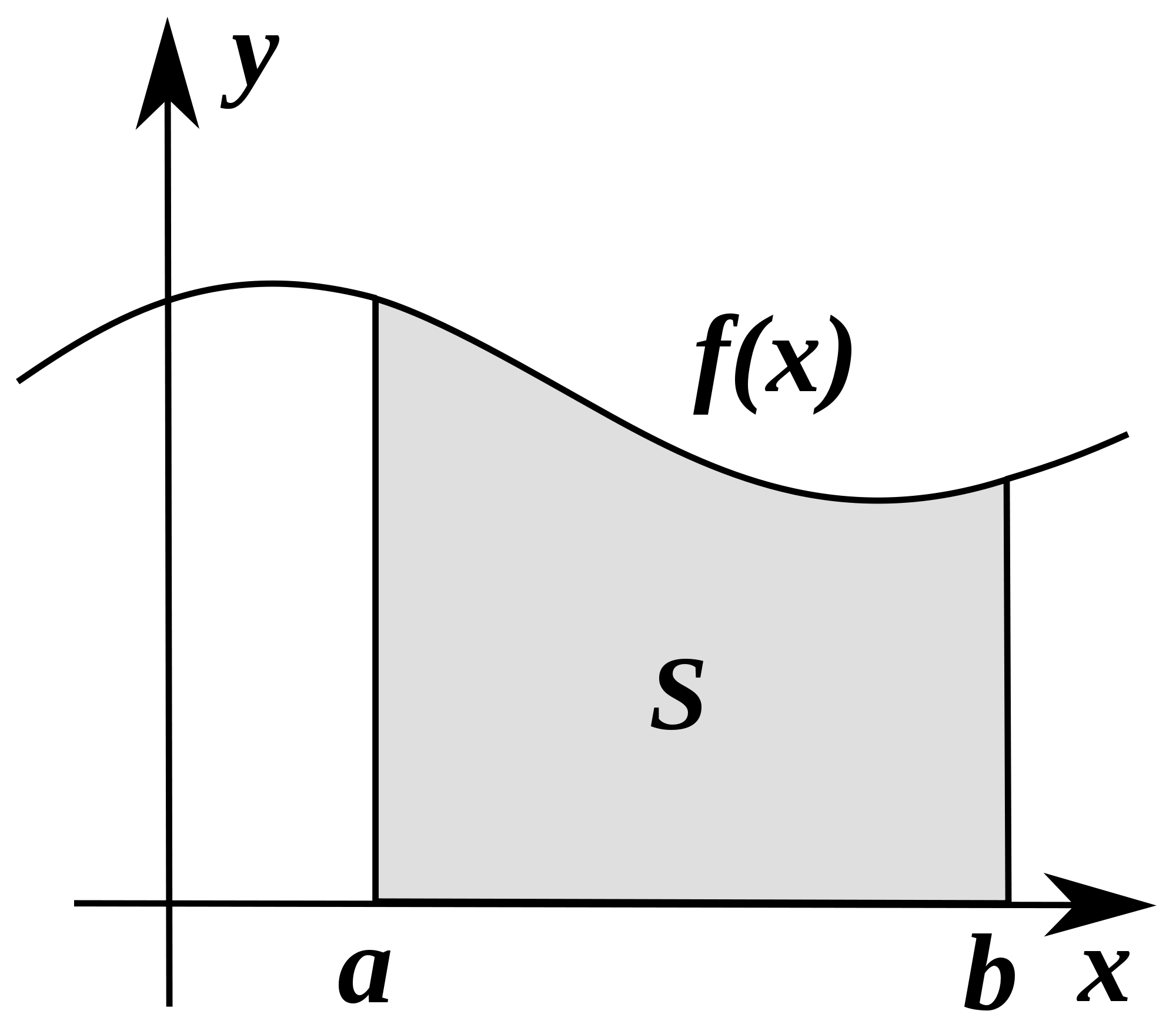# Definite Integral Challenge

Calculus Level 3$\large \int_{0}^{2}\frac{\left ( 16-x^2 \right )x}{16-x^2+\sqrt{\left ( 4-x \right )\left ( 4+x \right )\left ( 12+x^2 \right )}}\, dx$

The integral above has a simple closed form. Find this closed form.

Give your answer to 3 decimal places.

×

Problem Loading...

Note Loading...

Set Loading...